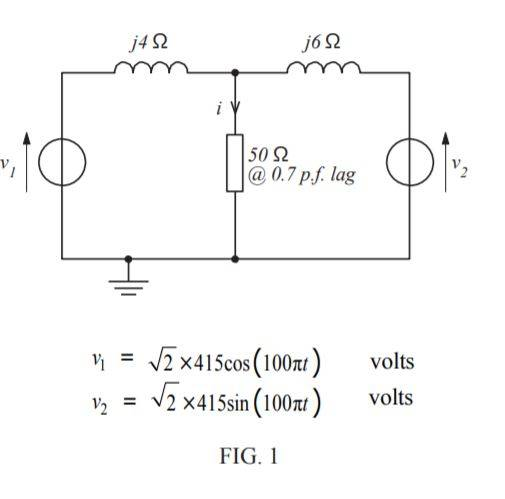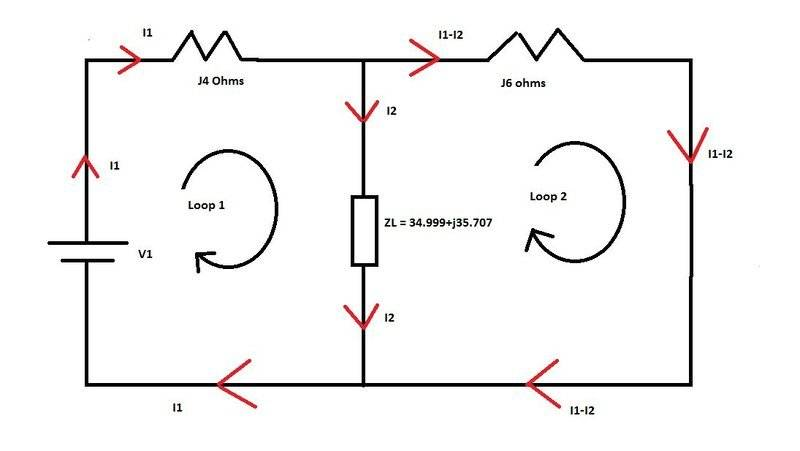# Superposition Theorem with complex numbers

• pgetts
In summary, Kirchhoff's voltage law is used to find the current in a load that is being supplied by two voltage sources with associated reactances. The superposition principle is then used to find the currents in the individual loops. Cramers rule is then used to find the sum of the currents in the individual loops. Finally, the attempt at a solution is to use the superposition principle to find the currents in the individual loops when the voltage sources are switched.)

#### pgetts

1. Homework Statement .
Figure 1 shows a 50 Ω load being fed from two voltage sources via their associated reactances. Determine the current i flowing in the load by:
(a) Thevenin's theorem
(b) Superposition
(c) Transforming the two voltage sources and their associated reactances into current sources( and thus forming a pair of Norton genoratorsV1 = √2 x 415cos(100pit) ∴ 415∠90 RMS
∴ j415 as cos leads sin by 90 degress.
V2 = √2 x 415sin(100pit) ∴ 415∠0 RMS
∴ 415
ZL = 50Ω @ 0.7 P.F ... convert into polar
sin-1 0.7 = 45.573 degrees
∴ 50∠45.573 convert into rectangular.
∴ 34.999+j35.707

2. Homework Equations
Kirchhoffs voltage law.
Superposition
Cramers Rule

3. The Attempt at a Solution

So, so far my process is to discard one of the voltage sources (V2)
Work out the two loop equations and using V1 and then using Cramers Rule I get the below matrix's (Δ, Δ1, Δ2)

Δ
Loop 1 = -j4(I1) -35.002+j35.705(I2) = -j415 eq 1
Loop 2 = -j6(I1) 35.002+j41.705(I2) = 0 eq 2

Now following Cramers rule I get Δ= (-j4)(35.002+j41.705) - (-j6)(-35.002+j35.705)
Δ= (166.82-j140.008)-(214.23+j210.012)
Δ= -47.41-j350.02

Δ1 Matrix
-j415 -35.002+j35.705
0 35.002+41.705

Δ1 = -14525.8-j17307.6

Δ2 Matrix
-j4 -j415
-j6 0

Δ2=2490

And I2 would equal Δ2/Δ

And so on for the whole process again for the second voltage.

Then sum I2 from voltage source one minus I2 from voltage source 2.

My problem is my superposition is not correct and I cannot see where my mistake is.
I'm confident that my Thevenin's is correct. 5.714+j0.892A or 5.783∠8.872 Deg's as I also have this as my answer using Nortons.

#### Attachments

Last edited by a moderator:
What component of load current do you attribute to source v1? Your numerical value.
And to v2?

I'm getting I2 for V1 as

Δ2/Δ
2490/(-47.41-j350.2) = -0.946+j6.986)

I've

pgetts said:
Loop 1 = -j4(I1) -35.002+j35.705(I2) = -j415
This isn't right. Even after you fix the missing parentheses I think it still won't be right. Mark on your diagram the complete path followed by currents I1 and I2.

Look at your first equation. The current I1 only multiplies j4, but I1 also flows through the load so there should be a term where I1 multiplies the load impedance. Also, why is the term where I1 multiplies j4 negative?

Thanks guys. OK, so here is how I have distributed my currents for voltage source 1. As I move from the top of V1 for loop 1 the first component I encounter is the j4 ohm impedance. As this is with the current flow it would cause a voltage drop so my first component is -j4I1. The next component I encounter is the Load impedance which as it is with the current flow causes a voltage drop so this should give me -34.999+j35.707I2. The next component in the circuit is the voltage source. As this goes from negative to positive this would give me a voltage rise of +j415.
This gives me -j4(I1) -34.999+j35.707(I2) + j415= 0
-j4(I1) - 34.999+35.707(I2) = -j415 Loop 1 equation

For Loop 2 I start at the left hand side of the j6 ohm impedance and as it is with the current flow it would be a voltage drop which gives me -j6(I1-I2). Then following it round to the load impedance I am against the current flow which would give me a voltage rise which gives me +34.999+j35.707 so my loop 2 equation should read -6(I1-I2) +34.999+35.707(I2)
-6(I1) +6(I2) + 34.999+35.707 ...now add the I2's
-6(I1) +34.999+41.707(I2) = 0 Loop 2 equation

Final Loops for V1

-4(I1) - 34.999+35.707(I2) = -j415 eq 1
-6(I1) + 34.999+41.707(I2) = 0 eq 2#### Attachments

pgetts said:
-4(I1) - 34.999+35.707(I2) = -j415 eq 1
-6(I1) + 34.999+41.707(I2) = 0 eq 2

Your first equation (and in the second it wouldn't hurt) needs some parentheses like this:

-4(I1) - (34.999+35.707)(I2) = -j415 eq 1

And you've totally lost your imaginary constant j where needed:

-j4(I1) - (34.999+j35.707)(I2) = -j415 eq 1
-j6(I1) + (34.999+j41.707)(I2) = 0 eq 2

What do you get if you solve these?

•NascentOxygen
The Electrician said:
Your first equation (and in the second it wouldn't hurt) needs some parentheses like this:

-4(I1) - (34.999+35.707)(I2) = -j415 eq 1

And you've totally lost your imaginary constant j where needed:

-j4(I1) - (34.999+j35.707)(I2) = -j415 eq 1
-j6(I1) + (34.999+j41.707)(I2) = 0 eq 2

What do you get if you solve these?

O.k so using Cramer's Rule.

Δ = -j4(I1)x(34.999+j41.707)(I2) - (-j6)(I1)x(-34.999+j35.707)(I2) = (-47.414 - j349.99)

ΔI1 = (-j415)x(34.999+j41.707)(I2) - (0) x (-34.999+j35.707)(I2) = (17308.405-j14524.585)

ΔI2 = (-j4)(I1)x(0) - (-j6)(I1) x (-j416) = (2490)

I1 = ΔI1/Δ = (17308.405-j14524.585) / (-47.414-j349.99)

I1 = (34.1732+j54.0835)

I2 = Δ2/Δ = (2490) / (-47.414 - j349.99)

I2 = (-0.946447+j6.98627)

pgetts said:
O.k so using Cramer's Rule.

Δ = -j4(I1)x(34.999+j41.707)(I2) - (-j6)(I1)x(-34.999-j35.707)(I2) = ?

ΔI1 = (-j415)x(34.999+j41.707)(I2) - (0) x (-34.999-j35.707)(I2) = ?

You have sign errors involving the same quantity where you made the parentheses mistake (corrections in red). Try the calculations again.

The Electrician said:
You have sign errors involving the same quantity where you made the parentheses mistake (corrections in red). Try the calculations again.

Δ = -j4(I1)x(34.999+j41.707)(I2) - (-j6)(I1)x(-34.999-j35.707)(I2) = (381.07-j349.99)
ΔI1 = (-j415)x(34.999+j41.707)(I2) - (0) x (-34.999-j35.707)(I2) = (17308.4-j14524.6)
ΔI2 = (-j4)(I1)x(0) - (-j6)(I1) x (-j416) = (2490)

I1 = (17308.4-j14524.6)/ (381.07-j349.99) = (3.963+j3.639)
I2 = (2490)/ (381.07-j349.99) = (3.544+j3.255)

Thanks guy's I've got the right answer now. Simple mistake of not adjusting the sign on the j constant as I dropped a voltage across the impedance.

pgetts said:
Δ = -j4(I1)x(34.999+j41.707)(I2) - (-j6)(I1)x(-34.999-j35.707)(I2) = (381.07-j349.99)
ΔI1 = (-j415)x(34.999+j41.707)(I2) - (0) x (-34.999-j35.707)(I2) = (17308.4-j14524.6)
ΔI2 = (-j4)(I1)x(0) - (-j6)(I1) x (-j416) = (2490)

I1 = (17308.4-j14524.6)/ (381.07-j349.99) = (3.963+j3.639) should be (43.627 + j1.953)
I2 = (2490)/ (381.07-j349.99) = (3.544+j3.255)

Thanks guy's I've got the right answer now. Simple mistake of not adjusting the sign on the j constant as I dropped a voltage across the impedance.

Even though I1 doesn't figure into the final calculation of the current through the load, you've made a mistake in calculating its value.

Thanks. Yes must make sure that what I input into my calculator is correct. So just to confirm that with an impedance in the form of (a+bi), as the current crosses the impedance this would cause the voltage drop and both the real and imaginary parts sign to change i.e (-a-bi)